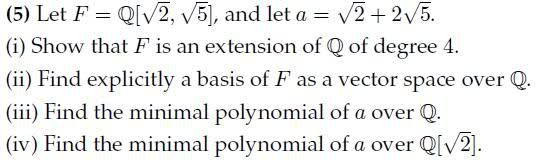# Number theory Extensions

FirepandaWouldn't mind a hint on how to start part iii), thanks.

edit: in my notes i have for a similar question:

'L=Q(20.5, 30.5)
F=Q(60.5)

degree of the min polynomial = 2, because L=F(a) and [L:F]=2
' (a = alpha)

Could someone clarify what L=F(a) means so I can understand the example? Thanks

Last edited:

Firepanda
Gold Member
The answer to the question in your second post is that you may use either expression for the reason you gave ("... both give you a poly of degree 4"). In fact, they give you the same poly. Does that clear up your original question?

Petek

Firepanda
The answer to the question in your second post is that you may use either expression for the reason you gave ("... both give you a poly of degree 4"). In fact, they give you the same poly. Does that clear up your original question?

Petek

Do they give the same poly?

If I do it that way I get

u - 20.5 = i

u2 - 2u20.5 - 2 = -1

u2 - 1 = 2u20.5

u4 - 2u2 + 1 = 8u2

u4 - 10u2 + 1 = 0

u4 - 2u2 + 9 = 0

Hence I have two different polynomials for the question I'm trying to do, both degree 4 and monic and I'm not sure which to choose.

Gold Member